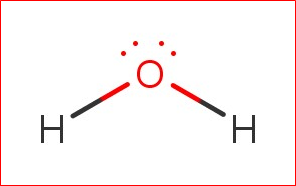# Problem: Explain why the HOH molecule is bent, whereas the HBeH molecule is linear.

###### FREE Expert Solution

The possible molecular geometries are:For HOH:

H can't be the central atom → O is the central atom.

Total valence electrons:

Group             Valence Electrons

H         1A                   2 × 1 e2 e

O         6A                   1 × 6 e6 e

Total:  8 valence e

Lewis structure:89% (95 ratings)###### Problem Details

Explain why the HOH molecule is bent, whereas the HBeH molecule is linear.

Frequently Asked Questions

What scientific concept do you need to know in order to solve this problem?

Our tutors have indicated that to solve this problem you will need to apply the Electron Geometry concept. You can view video lessons to learn Electron Geometry. Or if you need more Electron Geometry practice, you can also practice Electron Geometry practice problems.

What professor is this problem relevant for?

Based on our data, we think this problem is relevant for Professor Rabinovich's class at UNC.

What textbook is this problem found in?

Our data indicates that this problem or a close variation was asked in Chemistry - OpenStax 2015th Edition. You can also practice Chemistry - OpenStax 2015th Edition practice problems.